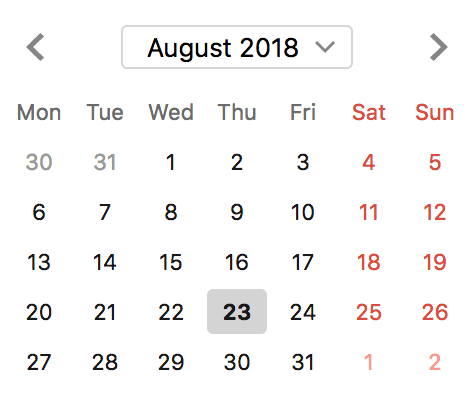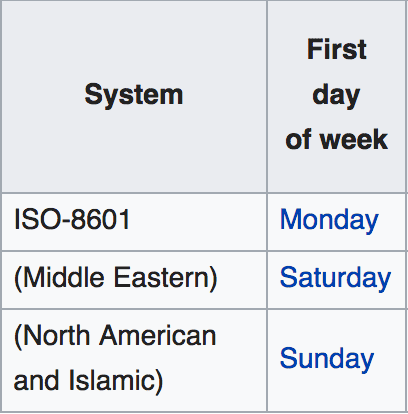# Building a calendar UI in ELM - Part 1

August 26, 2018

ELM was redesign recently and `Date` and `Time` have been removed from the core to the `Time` library. With that in mind let’s build a calendar.

Note: In the following some code samples are heavily influenced or copied from elm-community/elm-datepicker.

In this first part, we’ll make a list of dates for a give month and year. Eg: [1 Jan 2018, 2 Jan 2018,.., 31 Jan 2018]. I’ll skip a lot of steps but you’ll find the whole code attached at the end of this article.

## Get now’s Posix and here’s Zone

To start we’ll need to get the current time in the form of a `Posix`, the user’s zone and save it the `Model`.

``````type alias Model =
{ now : Time.Posix
, here : Time.Zone
}``````

We’ll get those information at initialisation time by running this task:

``````Task.map2 Tuple.pair Time.here Time.now

## Turn the Posix and Zone into a human friendly Date

So now that we have the user’s `Zone` and `Posix` in the model we can try to get the first and last day of the month. The problem is that `Posix` is the number of milliseconds elapsed since some arbitrary moment, so we’ll need to transform this `Posix` into a humanly understandable date (with years, month, days,..). Let’s make a `toDate`:

``````type alias Date =
{ year : Int
, month : Time.Month
, day : Int
, weekDay : Time.Weekday
, hour : Int
, minute : Int
, seconds : Int
, milliseconds : Int
, posix : Time.Posix
, zone : Time.Zone
}

toDate : Time.Zone -> Time.Posix -> Date
toDate zone time =
{ year = Time.toYear zone time
, month = Time.toMonth zone time
, day = Time.toDay zone time
, weekDay = Time.toWeekday zone time
, hour = Time.toHour zone time
, minute = Time.toMinute zone time
, seconds = Time.toSecond zone time
, milliseconds = Time.toMillis zone time
, posix = time
, zone = zone
}``````

## Get the first and last day of the month

To make the list of days we’ll need to get the edges, the first and last day of the month.

To start let’s get the first day of the month. To do so we’ll need to:

1. calculate the number of days between the current date and the first of the month;
2. change the result to milliseconds;
3. add it to the now `Posix`.
``````changeDay : Int -> Time.Zone -> Time.Posix
changeDay day zone time =
let
date =
toDate zone time
{-(1)-}
dayDiff =
day - date.day

{-(2)-}
dayDiffInMillis =
dayDiff * millisecondsInDay
in
{-(3)-}
(Time.posixToMillis date.posix + dayDiffInMillis)
|> Time.millisToPosix

millisecondsInDay : Int
millisecondsInDay =
1000 * 60 * 60 * 24

changeDay 1 model.here model.now``````

Let’s get the last day of the month now. We could use the `changeDay` function but we don’t know how many days are in the current month. We’ll need to create a function `daysInMonth : Int -> Month -> Int` which will take a year and month return a number of days. Nothing fancy, just mapping the month to the number of days, the only challenge will be to return the right amount for the month of `February` (accounting for leap year).

I will just share the `isLeapYear : Year -> Bool` function, where `Year` is an `Int`.

``````isLeapYear : Year -> Bool
isLeapYear y =
modBy y 400 == 0 || modBy y 100 /= 0 && modBy y 4 == 0``````

This code was copied from elm-community/elm-time.

Then we’ll just need to call `changeDay (daysInMonth date.year date.month) date` to get the last date of the current month.

## Get the first and last date of the calendar grid

The calendar is going to be a grid of 7 columns and a variable amount of rows. Eg:At the moment we've got the first (Wed 1 Aug 2018) and the last day (Fri 31 Sep 2018) of the month. We'll need to extend these dates to reach the first and last day of the week.

But what should be the first day of the week? Here is what Wikipedia says:We’re going to need to have the first day of the week configurable.

``````type alias Config =
{ firstDayOfWeek : Time.Weekday }

config : Config
config =
{ firstDayOfWeek = Mon }``````

From there we should be able to get the last day of the week with a simple mapping function, `previousWeekday: Time.Weekday -> Time.Weekday`.

Right, so how do we go from the first day of the month to the first date of the calendar (Mon 30 Aug 2018) (I won’t go over how to get the last date of the calendar since it’s a very similar technique)?

Let’s go over the principle first and we’ll see the implementation after.

We start with our first date of the month then we want to subtract a day until we reach the first date of the calendar. Eg:

• Wed 1 Aug 2018

• is it Mon? No
• Tue 31 Jul 2018

• is it Mon? No
• Mon 30 Jul 2018

• is it Mon? Yes. Stop

Let’s see the implementation:

``````firstDateOfWeek : Time.Weekday -> Time.Zone -> Time.Posix -> Time.Posix
firstDateOfWeek firstWeekday zone time =
let
cond newTime =
firstWeekday == .weekday (toDate zone newTime)

rec newTime =
if cond newTime then
newTime
else
(Time.posixToMillis newTime - millisecondsInDay)
|> Time.millisToPosix
|> rec
in
rec time``````

We now got the first and last date of the calendar. We’ll use the same technique as before but with an accumulator.

``````dateEq : Time.Zone -> Time.Posix -> Time.Posix -> Bool
dateEq zone timeA timeB =
let
dateA =
toDate zone timeA

dateB =
toDate zone timeB
in
( .day dateA, .month dateA, .year dateA ) == ( .day dateB, .month dateB, .year dateB )

datesInRange : Time.Zone -> Time.Posix -> Time.Posix -> List Time.Posix
datesInRange zone firstDate lastDate =
let
rec acc newTime =
if dateEq zone newTime lastDate then
acc
else
(Time.posixToMillis newTime + millisecondsInDay)
|> Time.millisToPosix
|> (\t -> rec (acc ++ [ t ]) t)
in
rec [ firstDate ] firstDate``````

That’s it for now! We’ve got all the days in the calendar. In the next part we’ll use this list to render the calendar’s grid.

Here’s the result in a page and here is the code.

This is a multiple part tutorial:

• [Part 2][part_2]

[part_2]: {% post_url 2018-09-04-building-a-calendar-ui-in-elm-part-2 %}